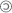## Departamento de Bioquímica, Genética e Inmunología Área de Genética. Universidad de Vigo

### Myriads: p-value-based dependence detection, simulation, and multiple testing correction

Rationale of the SGoF approach

SGoF test controls FWER (FamilyWise Error Rate) in the weak sense. FWER control means that what is aimed to be controlled is the probability of committing any (one or more than one) type I error in families of (simultaneous) comparisons. Weak control means that the FWER is maintained below a given error level under the complete null hypothesis (all nulls are true). Given a number S of tests, in Bonferroni technique the error rate per comparison is fixed to α/S which warrants strong FWER control (i.e. under all configurations of the true and false hypotheses). Therefore the per-comparison error rate diminishes with the number of tests. The problem here is that the power of each test also depends on the significance level. With very stringent significance level we will have very low power. SGoF method just performs an exact binomial test onto the expected (γ*S) and the observed proportion of tests with p-values below γ. The binomial test is performed at the α level. By default SGoF uses γ=α.

Importantly, the SGoF per comparison error rate (PCER) is proportional to a factor that increases with the number of tests resolving in this way the trade-off between type I error and statistical power. We can use the Myriads simulation tool to estimate, under a given correlation structure, the PCER in the worst case.

Alternatively, the false discovery rate (FDR) based methods as the Benjamini-Hochberg (BH), aim to control the proportion of false positives among the total ones (i.e. the proportion of the rejected null hypotheses which are erroneously rejected). Therefore, FDR methods suppose an important improvement compared to Bonferroni allowing a substantial gain in power. However, such methods are strongly dependent on the magnitude of the deviations of the alternative hypotheses from the null one, the relative frequency of false hypothesis and on the sample size. When deviations are weak or intermediate and the number of effects (for example number of genes under selection, number of significant protein interactions from a proteome, etc) are relatively low, the power of Benjamini-Hochberg method under biological sample sizes is low and diminishes as the number of tests becomes higher.

Thus, if no strong dependence is found in the data, SGoF is an interesting strategy for multiple testing adjustment when working with high-dimensional biological data. If strong dependence is present in the data, the SGoF PCER can be estimated by simulation in order to have a conservative guess on the number of true positives. In addition, the more robust methods to dependency as SLIM and Bon-SEV (Bon-EV modified to incorporate SLIM's π0 estimate) can be considered.

SGoF is calculated by an exact binomial test when the number of tests is lower than 10 and a G test with the Williams' correction in any other case.

Carvajal-Rodriguez A (Bioinformatics 34: 1043-104, 2018) [DOI]
Carvajal-Rodriguez A, de Uña-Alvarez J (PLoS ONE 6(9): e24700, 2011) [Journal Link]
AP. Diz et al (Molecular & Cellular Proteomics 10: M110.004374, 2011) [Online early]
Carvajal-Rodríguez et al (BMC Bioinformatics 10:209, 2009) [PDF]

Computation of the proportion of true nulls

When computing the q-values it is necessary to estimate the proportion of true null hypotheses (π0). There are different methods to do so and the Myriads software incorporates some of them. The methods are:

• Bootstrap (B): The bootstrap method (Storey et al., 2004).

• SDPB (D): The standard deviation proportional bounding method (Meinshausen and Rice, 2006)

• LBE (L): Location Based Estimator (Dalmasso et al., 2005).

• Smooth (S): Cubic Spline method (Storey & Tibshirani, 2003).

• Histogram (H): The histogram based method (Nettleton, et al., 2006).

• Median (ZG): The median based method (Zhang and Gant, 2004).

By default, Myriads performs all these methods and computes π0 as the mathematical mode of them. The mode is computed grouping the values by intervals of 5%, beginning from the highest π0 value. If the set of π0's is multimodal the highest mode is selected. Alternatively, Myriads also permits to select as π0 the highest from the different computed values or just the value obtained by any of the methods.

Computation of q-values

Once the value of π0 is estimated the q-value of a given p-value p(i) is computed as:

min{π0*S*p(t) / (t*C)} for all p(t) ≥ p(i)
where S is the number of tests, i is the position of p(i) in the list of sorted p-values and C = 1.0 - (1.0-p(t))S if the robust option was selected or, on the contrary, C = 1 (by default).

Back | Contact |2017-2020 Antonio Carvajal Rodríguez. Last updated 25/09/2020